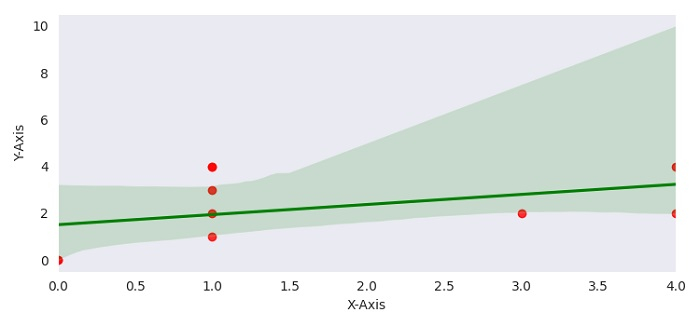# How to show different colors for points and line in a Seaborn regplot?

To show different colors for points and line in a Seaborn regplot, we can take the following steps −

• Set the figure size and adjust the padding between and around the subplots.

• Make a Pandas dataframe with key X-axis and Y-axis.

• Plot numeric independent variables with regression model.

• To display the figure, use show() method.

## Example

import pandas
import matplotlib.pylab as plt
import seaborn as sns
import numpy as np

plt.rcParams["figure.figsize"] = [7.50, 3.50]
plt.rcParams["figure.autolayout"] = True

df = pandas.DataFrame({"X-Axis": [np.random.randint(5) for i in range(10)], "Y-Axis": [np.random.randint(5) for i in range(10)]})
sns.regplot(x='X-Axis', y='Y-Axis', data=df, scatter_kws={"color": "red"}, line_kws={"color": "green"})

plt.show()

## Output